The HTSNP Procedure

### Diversity

Let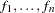represent the proportional frequencies of theunique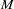-locus haplotypes in the input data set. The locus or allelic diversityfor theindividual loci and the overall haplotype diversity
can be calculated as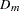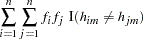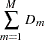where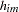is the allele of theth haplotype observed at theth locus and the indicator function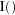equals 1 when true and 0 otherwise (Clayton 2002).

Based on a selected subset ofSNPs, theobserved haplotypes can be partitioned into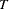distinct groups. Let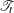represent the set of haplotypes in group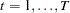, where each set contains all haplotypes that have identical alleles at theselected loci. The residual diversity is calculated by Clayton (2002) by summing the within-group diversity over thegroups, again both for the individual loci and over all haplotypes: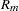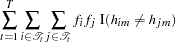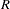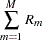where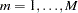. Note that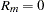if locusis one of theselected SNPs.

### Evaluating Sets of htSNPs

One of two criteria for finding the optimal set of htSNPs can be selected with the CRITERION= option. Using the diversity measures previously defined, the proportion of diversity explained (PDE) by a candidate SNP set can be calculated to evaluate the goodness of the set (Clayton 2002):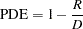The higher (that is, closer to 1) the value of PDE is, the better the set of htSNPs is for explaining the diversity among the haplotypes.

Alternatively, the approach of Stram et al. (2003) is implemented when CRITERION=RSQH. For these computations, define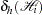to be the actual number of copies of haplotypethat an individual with the-locus haplotype pair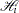(usually unknown) and genotype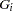carries. Assuming Hardy-Weinberg equilibrium, this can be estimated as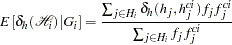where
is the set of haplotype pairs,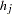and its complement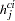, compatible with genotype. Then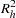can be defined as follows for each haplotype: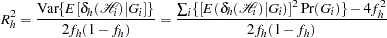withrepresenting each possible-locus genotype at the selected SNPs and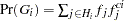. The set ofSNPs with the highest (that is, closest to 1) value of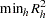is selected as the best set of htSNPs, for it optimizes the predictability of the common haplotypes (Stram et al. 2003).

Note: This procedure is experimental.Previous Page | Next Page | Top of Page